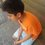# Can someone tell me why this happens?

I observed this awkward repetition while making a strategy for the game "odd-eve". Can someone tell me why this happens?

Let ${ a }_{ 1 }$ be the sum of digits of $x$ in base 10.

Let ${ a }_{ n}$ be the sum of digits of ${ a }_{ n-1}$ in base 10, for integers $n>1$.

Let $g\left( x \right) =\lim _{ n\rightarrow \infty }{ { a }_{ n } }$

I observed that:-

$g\left( 2 \right) =2$ $g\left( 4 \right) =4$ $g\left( 8 \right) =8$ $g\left( 16\right) =7$ $g\left( 32 \right) =5$ $g\left( 64 \right) =1$

then

$g\left( 128 \right) =2$ $g\left( 256\right) =4$ $g\left( 512 \right) =8$ $g\left( 1024 \right) =7$ $g\left( 2048 \right) =5$ $g\left( 4096 \right) =1$

and this pattern is repeating again and again.

$g\left( 8192 \right) =2$ $g\left( 16384 \right) =4$

and so on.........

So, The pattern for powers of 2 is $2,4,8,7,5,1,2,4,8,7,5,1$

and

The pattern for powers of 3 is $3,9,9,9,9,9,9,9,9,9,9,9$

The pattern for powers of 4 is $4,7,1,4,7,1,4,7,1,4,7,1$

The pattern for powers of 5 is $5,7,8,4,2,1,5,7,8,4,2,..$

Why does it work?

Does it work for powers of all integers?

Does it work in all bases?Note by Archit Boobna
6 years, 1 month ago

This discussion board is a place to discuss our Daily Challenges and the math and science related to those challenges. Explanations are more than just a solution — they should explain the steps and thinking strategies that you used to obtain the solution. Comments should further the discussion of math and science.

When posting on Brilliant:

• Use the emojis to react to an explanation, whether you're congratulating a job well done , or just really confused .
• Ask specific questions about the challenge or the steps in somebody's explanation. Well-posed questions can add a lot to the discussion, but posting "I don't understand!" doesn't help anyone.
• Try to contribute something new to the discussion, whether it is an extension, generalization or other idea related to the challenge.
• Stay on topic — we're all here to learn more about math and science, not to hear about your favorite get-rich-quick scheme or current world events.

MarkdownAppears as
*italics* or _italics_ italics
**bold** or __bold__ bold
- bulleted- list
• bulleted
• list
1. numbered2. list
1. numbered
2. list
Note: you must add a full line of space before and after lists for them to show up correctly
paragraph 1paragraph 2

paragraph 1

paragraph 2

[example link](https://brilliant.org)example link
> This is a quote
This is a quote
    # I indented these lines
# 4 spaces, and now they show
# up as a code block.

print "hello world"
# I indented these lines
# 4 spaces, and now they show
# up as a code block.

print "hello world"
MathAppears as
Remember to wrap math in $$ ... $$ or $ ... $ to ensure proper formatting.
2 \times 3 $2 \times 3$
2^{34} $2^{34}$
a_{i-1} $a_{i-1}$
\frac{2}{3} $\frac{2}{3}$
\sqrt{2} $\sqrt{2}$
\sum_{i=1}^3 $\sum_{i=1}^3$
\sin \theta $\sin \theta$
\boxed{123} $\boxed{123}$

## Comments

Sort by:

Top Newest

Hint: $\bmod 9$, divisibility rule.

- 6 years, 1 month ago

Log in to reply

One line. That beautiful number $9$! +1

- 6 years, 1 month ago

Log in to reply

Thanks so much!

I got it. The sum of digits mod 9 is same as the number mod 9.

And 2^n mod 9 is repeating every few terms.

- 6 years, 1 month ago

Log in to reply

×

Problem Loading...

Note Loading...

Set Loading...